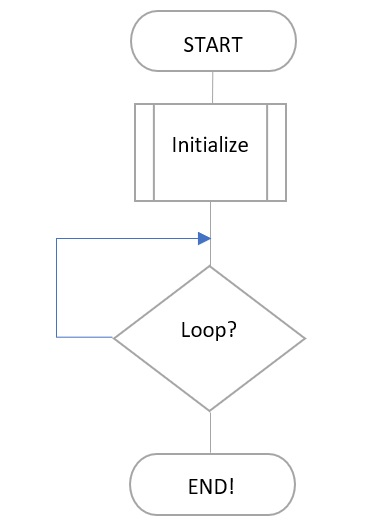## Document Information

 Document Title The “while” and “do while” statements Document Number & Revision 403 - 0003 - 1.0 Release Date April  12, 2019 Document Status Rev 1: Original Document

## Synopsis

With the early advent of programming, it was very quickly realized that a way of repeating a set of instructions was required. You could simply rerun the code over and over again, however for complex systems this would not be practical, especially where initialization of the system was required. The while statement is C is similar to the goto statement in old basic syntax, however somewhat more elegant and usefull.

Note
This topic is introductory, and should be read in conjunction with other topics in this series of training documents.

## Introduction

Looping through code is essential to any computerised system, where a set of instructions are repeatedly run and descisions made based on those instructions. To show a graphical representation of a loop, a simplified operation is shown in the diagram below. The system remains active and does not shut down or reach an EOL or end of line state and have nothing left to do. The code is structured in such a way that the code is always run.There are two types of while statements, the first is where the condition is checked before the code is run and the second is where the code is run and then the loop condition is checked. There is a key difference here, and it is important to note as it does determine which of the two should be used.

• while(condition) means that the code is run only if the condition is true
• do ... while(condition) means that the code is run regardless of whether the condition is true or not, so it runs atleast once before it is stopped or not run again

### Infinite loop

To implement the infinite loop, you may use either of the two options below. while(1){} and do {} while(1), provide the same result, ie the code will always loop even though while(1) checks the loop condition before hand and do while(1) checks the loop condition after the loop has run.

``` // First sample code while(1){} and do {} while(1), ```

### Conditional Loop

If the code were to say while(a), this means while a = 1 or true then loop. If the code were to say while(a==1), then this requires that the variable be initialized otherwise a would depend on the random nature of a PIC at startup. This can be precarious, as there is no way of knowing what the condition of the device will be. If for example a = 0, then in that condition the following code would never run

For example:

``` bit a; void main(){ while(a==1){ …run commands; } } ```

However, initialize a = 1 and the code runs for definite. It would be a best practice to initialize the variable especially for operation critical decisions.

``` bit a; void main(){ a=1; while(a==1){ …run commands; } } ```

The above code is essentially repeating itself. However, this setup can be used like an if statement. In the code below, when the device is initialized a is equal to one or true. However, during the course of operation the value of a can be changed to 0. The problem with the code below is that if it ever changes the back to 1, then the program essentially ends as the next command after a = 0 is nothing. You would have to reboot the system to start again. If that is then intention, then this is useful.

``` bit a; void main(){ a=1; while(a==1){ …run commands; } while(a==0){ …run commands; } } ```
The standard way of writing code that loops is shown below. An infinite loop is created, and within the loop descisions are made concerning the out come of each code path. See below:

``` bit a; void main(){ a=1; while(1){ // Loops indefinitely if(a==1) { …run commands; } if(a==0) { …run commands; } } } ```

The same would be true for the do...while:

``` bit a; void main(){ a=1; do{ // Loops indefinitely if(a==1) { …run commands; } if(a==0) { …run commands; } }while(1); } ```

## Conclusion

Both while statements have value depending on how or where they would be used. At times this may come down to a programming style or preference. It may also be based on when the condition is evaluated for the loop.